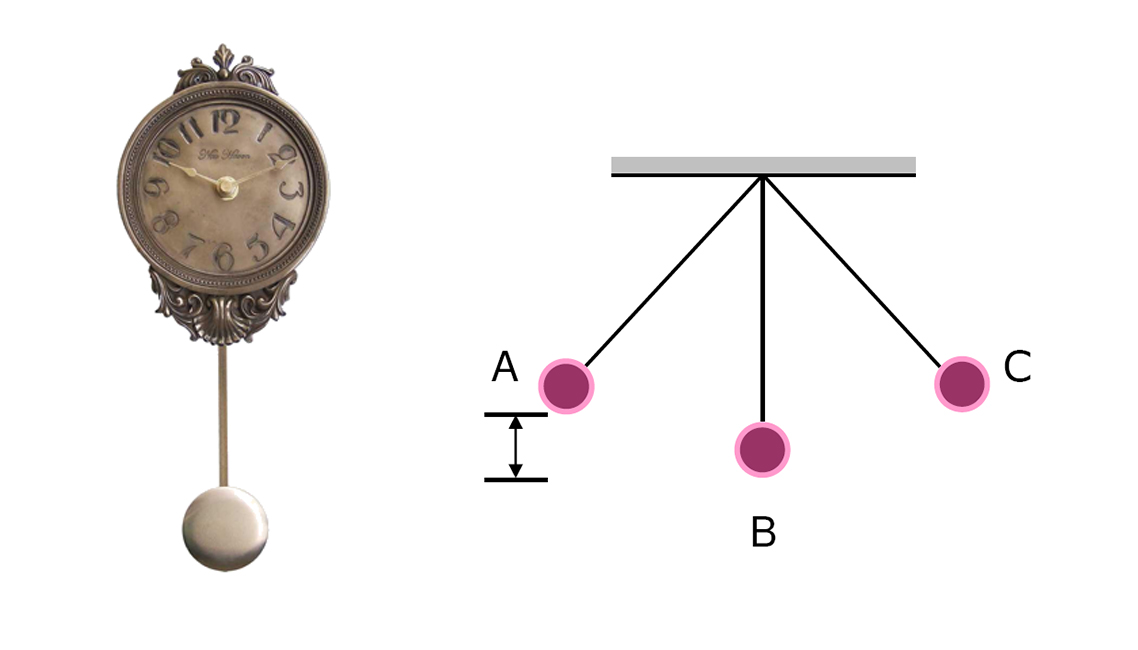# tick.. tick...a pendulum in the clock with a mass 500 grams is swing with the highest point A (h) 20 cm from first position B. if The acceleration of gravity is $-9.8 m/s^{2}$, velocity of pendulum in B point is? velocity in $m/s$

×